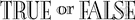# mathematical logic

(redirected from Mathematical logician)
Also found in: Thesaurus, Encyclopedia.
Related to Mathematical logician: formal logic

## mathematical logic

n
1. (Logic) symbolic logic, esp that branch concerned with the foundations of mathematics
2. (Mathematics) symbolic logic, esp that branch concerned with the foundations of mathematics
Collins English Dictionary – Complete and Unabridged, 12th Edition 2014 © HarperCollins Publishers 1991, 1994, 1998, 2000, 2003, 2006, 2007, 2009, 2011, 2014

## symbol′ic log′ic

n.
a modern development of formal logic employing a special notation or symbolism capable of manipulation in accordance with precise rules. Also called mathematical logic.
 Noun 1mathematical logic - any logical system that abstracts the form of statements away from their content in order to establish abstract criteria of consistency and validitylogical system, system of logic, logic - a system of reasoningBoolean algebra, Boolean logic - a system of symbolic logic devised by George Boole; used in computerspropositional calculus, propositional logic - a branch of symbolic logic dealing with propositions as units and with their combinations and the connectives that relate themfunctional calculus, predicate calculus - a system of symbolic logic that represents individuals and predicates and quantification over individuals (as well as the relations between propositions)modal logic - a system of logic whose formal properties resemble certain moral and epistemological conceptsfuzzy logic - a form of mathematical logic in which truth can assume a continuum of values between 0 and 1